Published

# ME 461 Final Project: Line Following Segway Robot

We built a line-following balancing robot that uses computer vision and IR sensors to follow a line and detect obstacles.

IntermediateShowcase (no instructions)102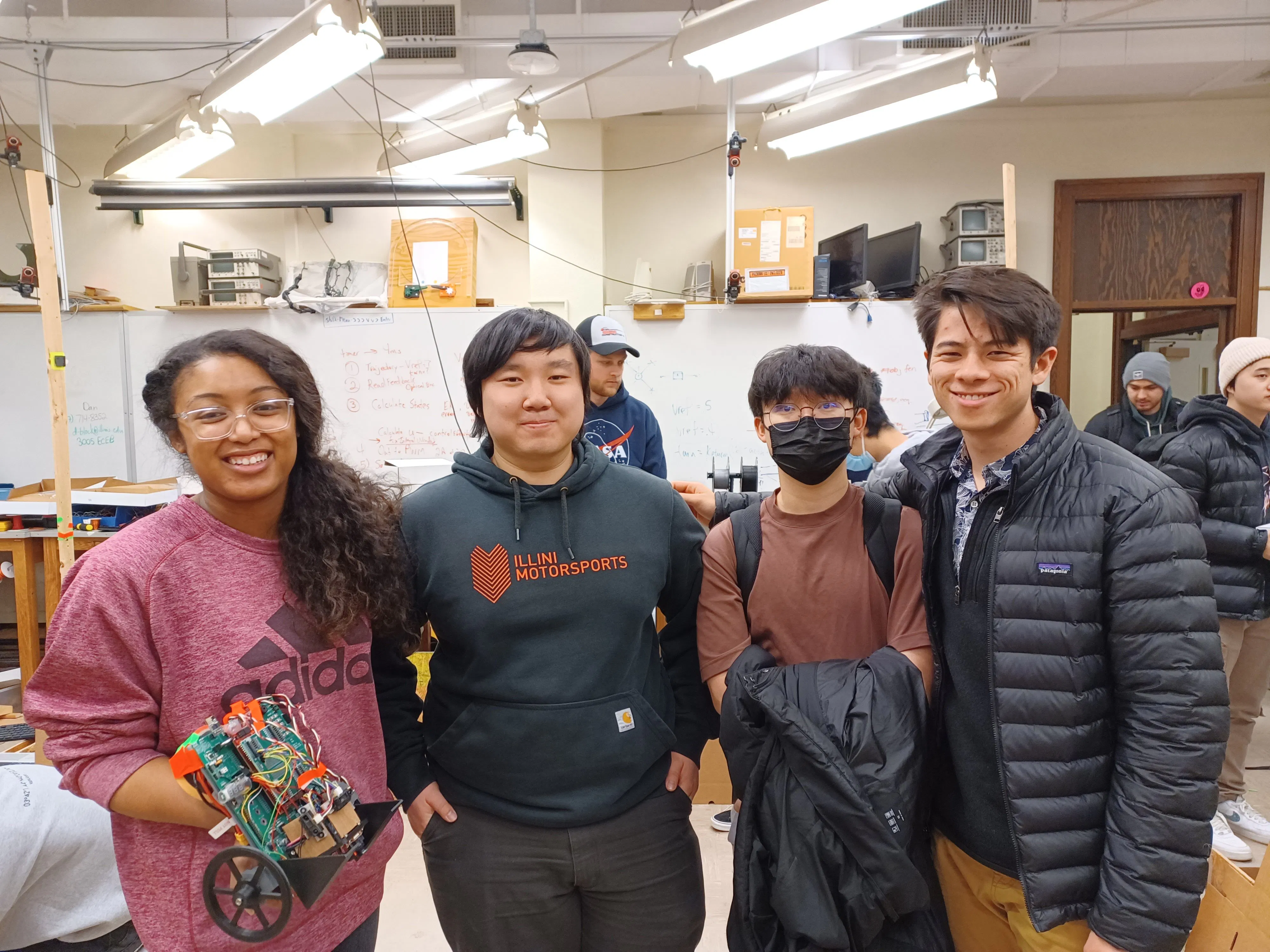## Things used in this project

### Hardware components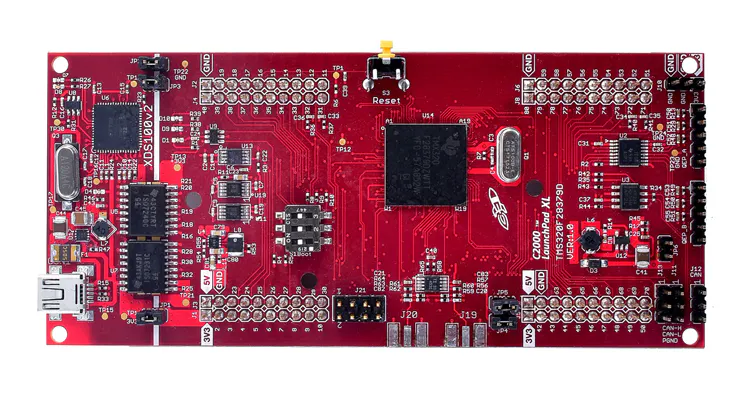Texas Instruments LAUNCHXL-F28379D C2000 Delfino LaunchPad
×1OpenMV Cam M7
×1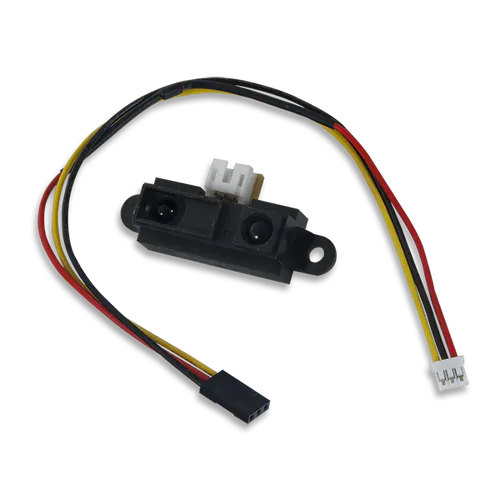Digilent IR Range Sensor
×1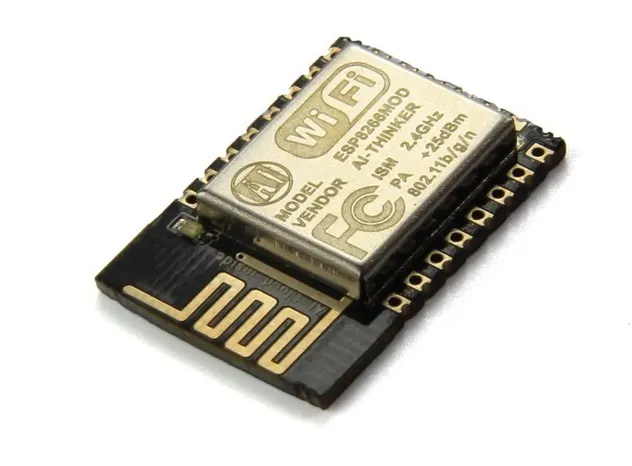Espressif ESP8266 ESP-12E
×1
 TDK Corporation InvenSense MPU-9250 3-Axis Accelerometer, Gyroscope & Magnetometer Sensor Module
×1

### Software apps and online servicesTexas Instruments Code Composer StudioLabVIEW Community Edition

## Custom parts and enclosures

### Camera Mount

STL of the 3D printed camera mount used to hold the OpenMV camera and IR sensors.

## Schematics

### IR Distance Sensor

Headers and connections needed for IR Distance Sensor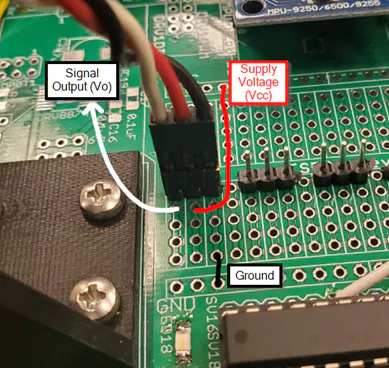### IR Distance Sensor to F28379D ADC

Connections from IR Distance Sensors to ADC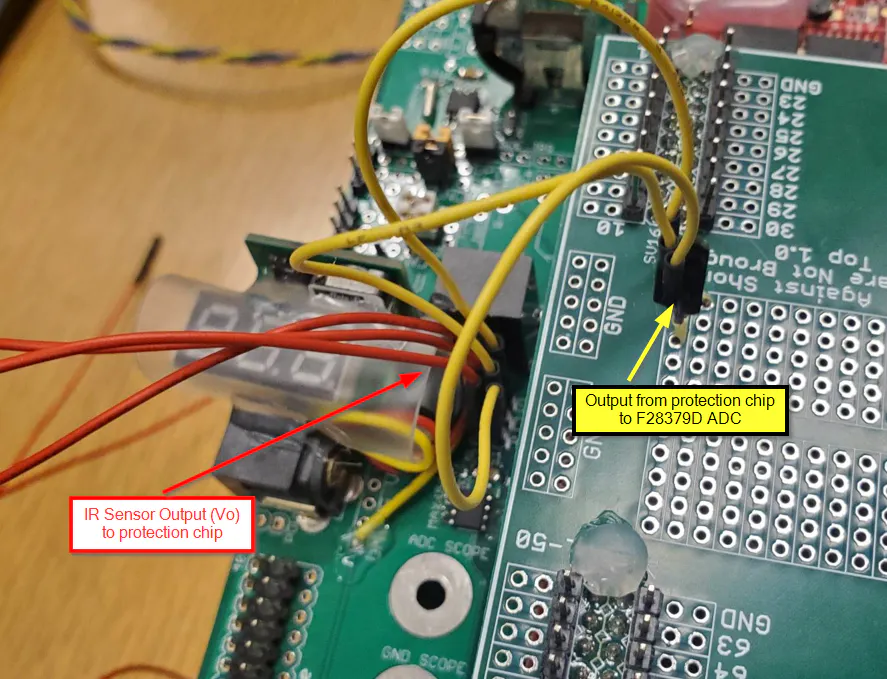## Code

### SegBotDynamics

MATLAB
Calculate gain matrices specific to your robot for slip balance control
```%% ME 461 Control Design of Mechanical Systems
% Final Project: Slip Balance Control of Segbot, a two wheel inverted pendulum
%
% Created by: Keona Banks
%
% Last Updated: 12/14/2022
%% Dynamic Model for Control Design
% Reference: <https://doi.org/10.1109/TCST.2019.2891522 https://doi.org/10.1109/TCST.2019.2891522>
%
% State Space Model:
%
% xdot = Ax*x+Bxu*u-Bxf*D*Bsf^-1*(As*x+Bsu*u)
%
% Slip Velocity:
%
% vsdot = As*x+Bsu*u - Bsf*D*Bsf^-1*(As*x+Bsu*u)
%
% where
%
% x = [tilt, tilt_rate, avg_rel_speed, diff_wheel_speed]'
%
% v = [right_vslip, left_vslip]'
%
% u = [avg_torque, diff_torque]'
%
% D = [right_slip_ratio, 0; 0, left_slip_ratio]

% Constants
m = .00003;     % Wheel mass [lb]
M = 2;          % Body mass [lb]
R = 2.3352/12;  % Wheel radius [ft]
L = 7.438/12;   % Body length
w = 6.811/12;   % Body width [ft]
d = 1/12;       % Body thickness [ft]
cent_len = L/2; % Center of body mass to center of wheel [ft]
br = 0.5/12;    % Right wheel thickness [ft] (This is a best guess, terms were not defined)
bl = 0.5/12;    % Left wheel thickness [ft] (This is a best guess, terms were not defined)
g = 32.2;       % Gravity acceleration [ft/s^2]

Ia = .5*m*R^2;    % Wheel axial inertia
Id = .25*m*R^2;   % Wheel diametrical inertia
Iy =(w^2+L^2)/12; % Body inertia in pitch
Iz =(w^2+d^2)/12; % Body inertia in yaw

% Paper did not define Izz and Iwd variables so I will assume:
Izz = Iz;
Iwd = Id;

Del = (Iz+2*Id)*(Iy*M+2*m*(Iy+M*cent_len^2))+(w^2/2)*Iy*M*m+w^2*m^2*(Iy+M*cent_len^2);
xi1 = g*cent_len*M*((2*Id+Iz)*(M+2*m)+(w^4/2*m*M)+w^2*m^2);
xi2 = 2*(M+2*m)*(Iz+2*Id)+2*m^2*w^2+w^2*m*M;
xi3 = M*m*cent_len*w^2/2+(Iz+2*Id)*M*cent_len;
xi4 = -g*cent_len^2*M^2*(m*w^2/2+2*Id+Iz);
xi5 = g*cent_len^2*M^2*(m*w^2/2+2*Id+Iz);
xi6 = 2*M*cent_len*(m*(br^2+bl^2)+(Izz+2*Iwd));
xi7 = 2*m*(Iy+M*cent_len^2)+M*Iy;
xi8 = m*(Iy+M*cent_len^2)*w^2/2+(Iy+M*cent_len^2)*(Iz+2*Id);

% Analytical Forms of System Matrices
Ax = [0 1 0 0
xi1/Del 0 0 0
xi1/Del 0 0 0
0 0 0 0];
Bxu = [0 0
xi2/Del 0
(xi2/Del+1/Ia) 0
0 1/Ia];
Bxf = [0 0
xi3/Del xi3/Del
(xi3/Del - R/(2*Ia)) (xi3/Del - R/(2*Ia))
-R/Ia R/Ia];
As = [xi4/Del 0 0 0
xi5/Del 0 0 0];
Bsu = [R/Ia-xi6/Del R/(2*Ia)
(R/Ia-xi6/Del) -R/(2*Ia)];
Bsf = [-R^2/Ia-((xi7*w^2)/(4*Del))-xi8/Del -xi8/Del+(xi7*w^2/(4*Del))
(-xi8/Del+(xi7*w^2)/(4*Del)) -R^2/Ia-(xi7*w^2)/(4*Del)-xi8/Del];
%% Computing Gains from Linear Matrix Inequalities(LMIs)
% There are 10 linear matrix inequalities to solve for the 4 gain matrices for
% all k<= j, k,j is {1,2,3,4}

% Term Coefficients
% D represents the slip ratios for the left and right wheel
% where traction = slip ratio * ideal traction
D1 = zeros(2,2); % total slip on both wheels
D2 = [1 0; 0 0]; % total slip on the left
D3 = [0 0; 0 1]; % total slip on the right
D4 = [1 0; 0 1]; % no slip

A1 = Ax-Bxf*D1*Bsf^-1*As;
A2 = Ax-Bxf*D2*Bsf^-1*As;
A3 = Ax-Bxf*D3*Bsf^-1*As;
A4 = Ax-Bxf*D4*Bsf^-1*As;

B1 = Bxu-Bxf*D1*Bsf^-1*Bsu;
B2 = Bxu-Bxf*D2*Bsf^-1*Bsu;
B3 = Bxu-Bxf*D3*Bsf^-1*Bsu;
B4 = Bxu-Bxf*D4*Bsf^-1*Bsu;

lambda = 15;  % Lambda >0 Parameter corresponds to the minimum bandwidth
% of the control system [Hz I assume]
% We sample every 1 ms
% There's a rule of thumb that sample time is 10 times smaller than time
% constant, therefore I chose 10 ms as the time constant
% rise time is roughly 2.3 times the time constant
% rise time is then 23 ms and if bandwidth is 0.35/rise time
% I'll say minimum bandwidth is roughly 15 Hz and go from there

% for j = 1:4
%     k=0;
%     while k<j
%         k=k+1;
%     end
% end

% Set LMI variables
% Where W = W' and W>0
% If there exist Y1,Y2,Y3,Y4 as 2 by 4 matrices that
% satisfy the LMIs then the sytem is stable by setting Kj = Yj*W^-1

% Initialize internal representation of the LMI system
setlmis([])
% Yk or Yj is a 2x4 rectangular matrix
Y1 = lmivar(2,[2,4]);
Y2 = lmivar(2,[2,4]);
Y3 = lmivar(2,[2,4]);
Y4 = lmivar(2,[2,4]);

% W is type 1 is a symmetric 4x4 matrix
W = lmivar(1,[4 1]);

% termID(1) left side is positive 1
% termID(2:3) [0,0] for outer factors [i,j] think just 1 1 in my case
% termID(4) LMI variable in the term
kj11 = newlmi;
lmiterm([kj11 1 1 W],A1+A1,1);
lmiterm([kj11 1 1 W],1,(A1+A1)');
lmiterm([kj11 1 1 -Y1],1,B1');
lmiterm([kj11 1 1 Y1],B1,1);
lmiterm([kj11 1 1 -Y1],1,B1');
lmiterm([kj11 1 1 Y1],B1,1);
lmiterm([kj11 1 1 W],4*lambda,1);

kj12 = newlmi;
lmiterm([kj12 1 1 W],A1+A2,1);
lmiterm([kj12 1 1 W],1,(A1+A2)');
lmiterm([kj12 1 1 -Y2],1,B1');
lmiterm([kj12 1 1 Y2],B1,1);
lmiterm([kj12 1 1 -Y1],1,B2');
lmiterm([kj12 1 1 Y1],B2,1);
lmiterm([kj12 1 1 W],4*lambda,1);

kj13 = newlmi;
lmiterm([kj13 1 1 W],A1+A3,1);
lmiterm([kj13 1 1 W],1,(A1+A3)');
lmiterm([kj13 1 1 -Y3],1,B1');
lmiterm([kj13 1 1 Y3],B1,1);
lmiterm([kj13 1 1 -Y1],1,B3');
lmiterm([kj13 1 1 Y1],B3,1);
lmiterm([kj13 1 1 W],4*lambda,1);

kj14 = newlmi;
lmiterm([kj14 1 1 W],A1+A4,1);
lmiterm([kj14 1 1 W],1,(A1+A4)');
lmiterm([kj14 1 1 -Y1],1,B1');
lmiterm([kj14 1 1 Y1],B1,1);
lmiterm([kj14 1 1 -Y4],1,B4');
lmiterm([kj14 1 1 Y4],B4,1);
lmiterm([kj14 1 1 W],4*lambda,1);

kj22 = newlmi;
lmiterm([kj22 1 1 W],A2+A2,1);
lmiterm([kj22 1 1 W],1,(A2+A2)');
lmiterm([kj22 1 1 -Y2],1,B2');
lmiterm([kj22 1 1 Y2],B2,1);
lmiterm([kj22 1 1 -Y2],1,B2');
lmiterm([kj22 1 1 Y2],B2,1);
lmiterm([kj22 1 1 W],4*lambda,1);

kj23 = newlmi;
lmiterm([kj23 1 1 W],A2+A3,1);
lmiterm([kj23 1 1 W],1,(A2+A3)');
lmiterm([kj23 1 1 -Y3],1,B2');
lmiterm([kj23 1 1 Y3],B2,1);
lmiterm([kj23 1 1 -Y2],1,B3');
lmiterm([kj23 1 1 Y2],B3,1);
lmiterm([kj23 1 1 W],4*lambda,1);

kj24 = newlmi;
lmiterm([kj24 1 1 W],A2+A4,1);
lmiterm([kj24 1 1 W],1,(A2+A4)');
lmiterm([kj24 1 1 -Y4],1,B2');
lmiterm([kj24 1 1 Y4],B2,1);
lmiterm([kj24 1 1 -Y2],1,B4');
lmiterm([kj24 1 1 Y2],B4,1);
lmiterm([kj24 1 1 W],4*lambda,1);

kj33 = newlmi;
lmiterm([kj33 1 1 W],A3+A3,1);
lmiterm([kj33 1 1 W],1,(A3+A3)');
lmiterm([kj33 1 1 -Y3],1,B3');
lmiterm([kj33 1 1 Y3],B3,1);
lmiterm([kj33 1 1 -Y3],1,B3');
lmiterm([kj33 1 1 Y3],B3,1);
lmiterm([kj33 1 1 W],4*lambda,1);

kj34 = newlmi;
lmiterm([kj34 1 1 W],A3+A4,1);
lmiterm([kj34 1 1 W],1,(A3+A4)');
lmiterm([kj34 1 1 -Y4],1,B3');
lmiterm([kj34 1 1 Y4],B3,1);
lmiterm([kj34 1 1 -Y3],1,B4');
lmiterm([kj34 1 1 Y3],B4,1);
lmiterm([kj34 1 1 W],4*lambda,1);

kj44 = newlmi;
lmiterm([kj13 1 1 W],A4+A4,1);
lmiterm([kj13 1 1 W],1,(A4+A4)');
lmiterm([kj13 1 1 -Y4],1,B4');
lmiterm([kj13 1 1 Y4],B4,1);
lmiterm([kj13 1 1 -Y4],1,B4');
lmiterm([kj13 1 1 Y4],B4,1);
lmiterm([kj13 1 1 W],4*lambda,1);

Wpos = newlmi;
lmiterm([-Wpos 1 1 W],1,1)

LMIs = getlmis;
[tmin,xfeas] = feasp(LMIs);
Y1_solve = dec2mat(LMIs,xfeas,Y1);
Y2_solve = dec2mat(LMIs,xfeas,Y2);
Y3_solve = dec2mat(LMIs,xfeas,Y3);
Y4_solve = dec2mat(LMIs,xfeas,Y4);
W_solve = dec2mat(LMIs,xfeas,W);

% The final gain matrices for the balance controller is
K1ns = Y1_solve*W^-1;
K2ns = Y2_solve*W^-1;
K3ns = Y3_solve*W^-1;
K4ns = Y4_solve*W^-1;
%% Pole Placement for the state observers
% The control method of slip detection estimates a slip ratio. Slip ratio =
% actual traction/ideal traction. We need an observer to estimate the actual traction
% since it is not directly measurable.

% state estimate z = [x fx] where x are the measured 4 states and fx is
% traction on the left wheel and traction on the right wheel

% z(k+1) = Az*zhat(k)+ Bz*u + L*(C*zhat(k)-x)

Az = [Ax Bxf
zeros(2,6)];
Bz = [Bxu
zeros(2,2)];
Cz = [eye(4,4) zeros(4,2)];

Dz = zeros(4,2);

% Is the system observable? Obsv should equal 6. Yes
Obsv = rank(obsv(Az,Cz));

sys = ss(Az,Bz,Cz,Dz);
check = pole(sys);

% placing negative real poles with two dominant ones
pp = [-2,-22,-22,-3,-4,-5];

% The observer gain is then a 6x4 matrix
L = place(Az',Cz',pp)';

% The discrete model boils down to
% zhat(k+1) = Az*zhat(k) + Bz*u(k) + L(C*zhat(k)-x)
%           = [Az+L*Cz]*zhat(k) +Bz*u(k) - L*x(k)
%           = E*zhat(k) + Bz*u(k) - L*x(k)

% zhat = [tilt tiltrate avg_rel_speed diff_wheel speed traction_r
% traction_l]
%% Final Matrices Needed for C Code Implementation

% Control Gain Matrices
K1ns
K2ns
K3ns
K4ns

% Coefficient Matrices for State Observer
E = Az+L*Cz
Bz
% State Observer Gain
L
% Ideal friction coefficient
M1 = -Bsf^-1*As
M2 = -Bsf^-1*Bsu
```

### SegBot Final Code

C/C++
`No preview (download only).`

### Line Following OpenMV Code

MicroPython
This program filters the OpenMV image, finds a best-fit line through the visible points, and uses PID to produce a steering value which is sent to the LaunchPad microcontroller over UART serial communication.
```BINARY_VIEW = True
GRAYSCALE_THRESHOLD = (240, 255)
MAG_THRESHOLD = 4
THETA_GAIN = 40.0
RHO_GAIN = 1.0
P_GAIN = 0.4 #0.7
I_GAIN = 0.7 # 0.0
I_MIN = -0.0
I_MAX = 0.0
D_GAIN = 0.1 # 0.1
STEERING_SERVO_INDEX = 0
THRESHOLD = (0, 50)
BINARY_VISIBLE = True

import sensor, image, time, math, pyb
from pyb import UART

uart = UART(3, 115200)

sensor.reset()
sensor.set_pixformat(sensor.GRAYSCALE)
sensor.set_framesize(sensor.QQVGA)
sensor.skip_frames(time = 2000)
clock = time.clock()

# Converts the position of the line to a theta value between [-1:1] and
# rho between -40 and 40
def line_to_theta_and_rho(line):
if line.rho() < 0:
if line.theta() < 90:
else:
else:
if line.theta() < 90:
if line.theta() < 45:
else:
else:

def line_to_theta_and_rho_error(line, img):
t, r = line_to_theta_and_rho(line)
r = r - (img.width() // 2)
return (t, r)

old_result = 0
old_time = pyb.millis()
i_output = 0
output = 0
last_vals =  * 10

while True:
clock.tick()
img = sensor.snapshot().binary([THRESHOLD]) if BINARY_VISIBLE else sensor.snapshot()

line = img.get_regression([(255, 255)], robust=True)
hist = img.get_statistics()
mean = hist.mean()
print_string = ""

if line and (line.magnitude() >= MAG_THRESHOLD): # Make sure line exists to avoid errors
img.draw_line(line.line(), color=127) # Draw line on image so we can tell what's going on

t, r = line_to_theta_and_rho_error(line, img) # Get initial theta and rho values
new_result = (t * THETA_GAIN) + (r * RHO_GAIN) # Multiply individual error by gains so we can tune
delta_result = new_result - old_result # Find difference in error
old_result = new_result # Keep track of K and K_1 for calculating derivative and integral of error

new_time = pyb.millis() # Current time
delta_time = new_time - old_time
old_time = new_time # Keep track of old time in order to calculate error derivative

p_output = new_result # Proportional value is just error
i_output = max(min(i_output + new_result, I_MAX), I_MIN) # Integral adds old error to integral counter
d_output = (delta_result * 1000) / delta_time # (New result - old result) / time step
pid_output = (P_GAIN * p_output) + (I_GAIN * i_output) + (D_GAIN * d_output)

output = 128 + max(min(int(pid_output), 90), -90)

if(abs(t) > 0.6): # line angle too extreme
uart.writechar(0)
print("Angle too steep - turn %d" % output)
elif (mean > 20): # too much noise
uart.writechar(0)
print("Noisy Image - turn %d" % output)
else: # line is good
uart.writechar(int(output))
print("Line Ok","Turn:",last_vals[-1],"Theta:",round(t,2),"Rho:",round(r,2))
else:
uart.writechar(0)
print("Line Lost - turn %d" % output)

last_vals[-1] = output
for i in range(1, len(last_vals)): #average last 10 values
last_vals[i-1] = last_vals[i]
```

## Credits

### Tyler Smithline

0 projects • 1 follower

### Richard Qiu

0 projects • 1 follower

### Keona Banks

0 projects • 1 follower

### Charley Zhao

0 projects • 0 followers
Thanks to Kwabena Agyeman.